# OR Function

Determines if any of the conditions in the test is TRUE

## What is the OR Function?

The OR Function is categorized under Excel LOGICAL functions. The function will determine if any of the conditions in a test is TRUE.

In financial analysis, the OR function can be useful in comparing two statements or two values. For example, A1 for either “a” or “b,” use =OR(A1=”a”,A1=”b”). The function can be used as the logical test inside the IF function to avoid extra nested IFs, and can be combined with the AND function.

### Formula

=OR(logical1, [logical2], …)

The function uses the following arguments:

1. Logical1 (required argument) – This is the first condition or logical value to evaluate.
2. Logical2 (optional argument) – The second condition or logical value to evaluate.

### How to use the OR Function in Excel?

As a worksheet function, it can be entered as part of a formula in a cell of a worksheet. To understand the uses of the function, let us consider an example:

#### Example 1

Let’s see how we can test a few conditions. Suppose we are given the following data: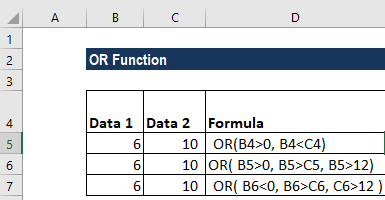We get the results below: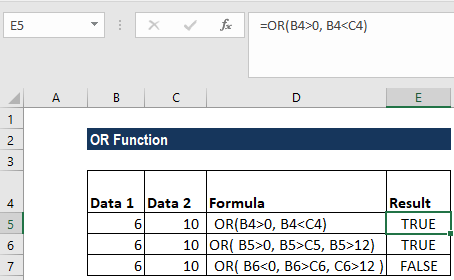In the above examples:

1. The function in cell E4 evaluates to TRUE, as BOTH of the supplied conditions are TRUE;
2. The function in cell E5 evaluates to TRUE, as the first condition, B5>0 evaluates to TRUE;
3. The function in cell E6 evaluates to FALSE, as ALL of the supplied conditions are FALSE.

#### Example 2

Let us now nest OR with IF. Suppose we are given student marks and we want the formula to return “PASS” if marks are above 50 in Economics or above 45 in Business Studies and “FAIL” for marks below it.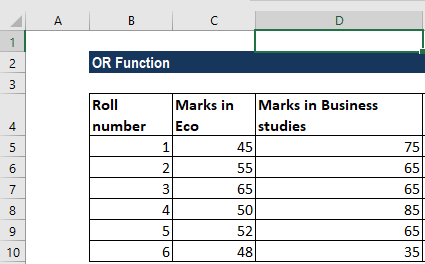The formula to use is: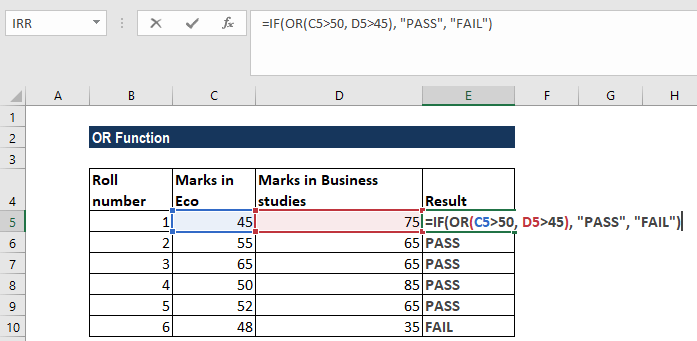We get the results below: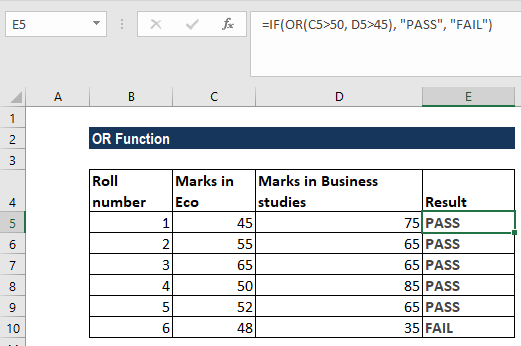Even if any one condition is satisfied, this function will return PASS.

#### Example 3

Suppose we want to highlight dates that occur on weekends. We can use the OR and WEEKDAY functions. Suppose we are given the data below: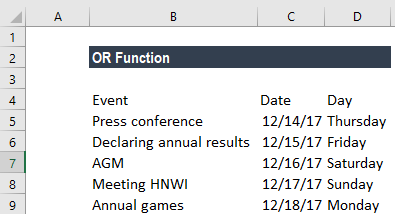Using conditional formatting, we can highlight cells that fall on weekends. For that, select the cells B2:B6 and in Conditional Formatting, we will put the formula =OR(WEEKDAY(B2)=7,WEEKDAY(B2)=1, as shown below: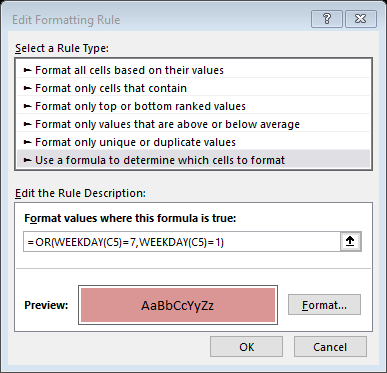We get the results below: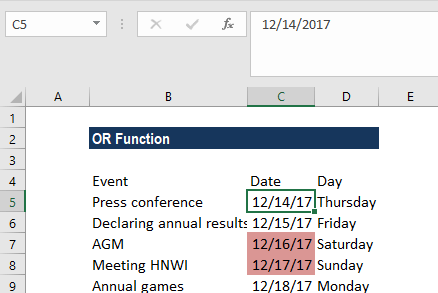This formula uses the WEEKDAY function to test dates for either a Saturday or Sunday. When given a date, WEEKDAY returns a number 1-7, for each day of the week. In their standard configuration, Saturday = 7 and Sunday = 1. By using the OR function, we used WEEKDAY to test for either 1 or 7. If either is true, the formula will return TRUE and trigger the conditional formatting.

If we wish to highlight the entire row, we need to apply the conditional formatting rule to all columns in the table and lock the date column. The formula to use will be =OR(WEEKDAY(\$B2)=7,WEEKDAY(\$B2)=1).

### A few things to remember about the OR Function:

1. We can use the function to test multiple conditions at the same time – up to 255 conditions in total.
2. #VALUE! error – Occurs if any of the given logical_test cannot be interpreted as numeric or logical values.
3. The function can also be used with the AND function, depending on the requirement.
4. If we enter OR as an array formula, we can test all values in a range against a condition. For example, the array formula will return TRUE if any cell in A1:A100 is greater than 15 ={OR(A1:A100>15}.
5. This function will ignore the text values or empty cells provided in the arguments.

Thanks for reading CFI’s guide to important Excel functions! By taking the time to learn and master these functions, you’ll significantly speed up your financial analysis. To learn more, check out these additional CFI resources:

• Excel Functions for Finance